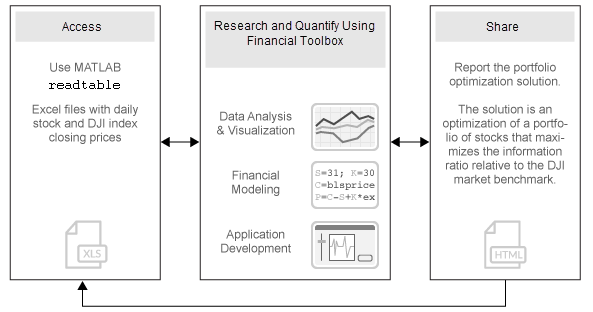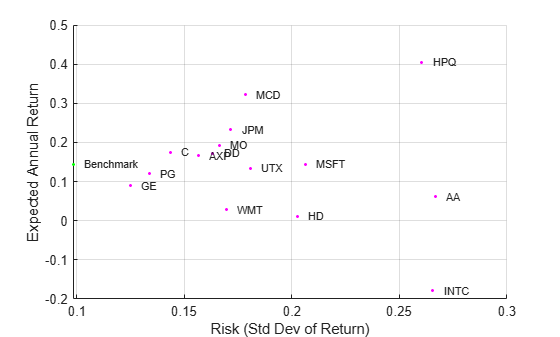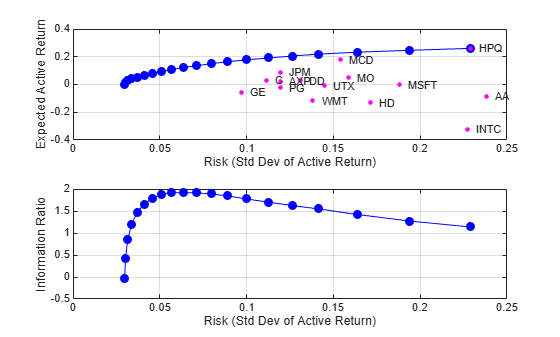Portfolio Optimization Against a Benchmark

This example shows how to perform portfolio optimization using the Portfolio object in Financial Toolbox™.

This example, in particular, demonstrates optimizing a portfolio to maximize the information ratio relative to a market benchmark. Specifically, financial data contained in a table is read into MATLAB® and visualizations (at both daily and annual levels) are performed. A Portfolio object is created with the market data using an active daily return for each asset. Using functions supporting a Portfolio object, the efficient frontier is calculated directly. Then, a customized optimization problem is solved to find the asset allocation with the maximized information ratio.Import Historical Data Using MATLAB®

Import historical prices for the asset universe and the Dow Jones Industrial Average (DJI) market benchmark. The data is imported into a table from a Microsoft® Excel® spreadsheet using the MATLAB® readtable function.

ans=10×32 table
Dates        DJI      AA       AIG      AXP      BA        C       CAT      DD       DIS      GE       GM       HD       HON      HPQ      IBM     INTC      JNJ      JPM      KO       MCD      MMM      MO       MRK     MSFT      PFE      PG        T       UTX      VZ       WMT      XOM
___________    _____    _____    _____    _____    _____    _____    _____    _____    _____    _____    _____    _____    _____    _____    _____    _____    _____    _____    _____    _____    _____    _____    _____    _____    _____    _____    _____    _____    _____    _____    _____

03-Jan-2006    10847    28.72    68.41    51.53    68.63    45.26    55.86    40.68    24.18     33.6    17.82    39.79    36.14    28.35    80.13    24.57    59.08    37.78    38.98    32.72    75.93    52.27    30.73    26.19    22.16    56.38     22.7    54.94    26.79     44.9    56.64
04-Jan-2006    10880    28.89    68.51    51.03    69.34    44.42    57.29    40.46    23.77    33.56     18.3    39.05    35.99    29.18    80.03     24.9    59.99    37.56    38.91    33.01    75.54    52.65    31.08    26.32    22.88    56.48    22.87    54.61    27.58    44.99    56.74
05-Jan-2006    10882    29.12     68.6    51.57    68.53    44.65    57.29    40.38    24.19    33.47    19.34    38.67    35.97    28.97    80.56    25.25    59.74    37.67     39.1    33.05    74.85    52.52    31.13    26.34     22.9     56.3    22.92    54.41     27.9    44.38    56.45
06-Jan-2006    10959    29.02    68.89    51.75    67.57    44.65    58.43    40.55    24.52     33.7    19.61    38.96    36.53     29.8    82.96    25.28    60.01    37.94    39.47    33.25    75.47    52.95    31.08    26.26    23.16    56.24    23.21    54.58    28.01    44.56    57.57
09-Jan-2006    11012    29.37    68.57    53.04    67.01    44.43    59.49    40.32    24.78    33.61    21.12    39.38    36.23    30.17    81.76    25.44    60.38    38.55    39.66    33.88    75.84    53.11    31.58    26.21    23.16    56.67     23.3     55.2    28.12     44.4    57.54
10-Jan-2006    11012    28.44    69.18    52.88    67.33    44.57    59.25     40.2    25.09    33.43    20.79    40.33    36.17    30.33     82.1     25.1    60.49    38.61     39.7    33.91    75.37    53.04    31.27    26.35    22.77    56.45    23.16    55.24    28.24    44.54    57.99
11-Jan-2006    11043    28.05     69.6    52.59     68.3    44.98    59.28    38.87    25.33    33.66    20.61    41.44    36.19    30.88    82.19    25.12    59.91    38.58    39.72     34.5    75.22    53.31    31.39    26.63    23.06    56.65    23.34    54.41    28.58    45.23    58.38
12-Jan-2006    10962    27.68    69.04     52.6     67.9    45.02    60.13    38.02    25.41    33.25    19.76    41.05    35.77    30.57    81.61    24.96    59.63    37.87     39.5    33.96    74.57    53.23    31.41    26.48     22.9    56.02    23.24     53.9    28.69    44.43    57.77
13-Jan-2006    10960    27.81    68.84     52.5     67.7    44.92    60.24    37.86    25.47    33.35     19.2    40.43    35.85    31.43    81.22    24.78    59.26    37.84    39.37    33.65    74.38    53.29     31.4    26.53    22.99    56.49    23.27     54.1    28.75     44.1    59.06
17-Jan-2006    10896    27.97    67.84    52.03    66.93    44.47    60.85    37.75    25.15     33.2    18.68    40.11    35.56     31.2    81.05    24.52    58.74    37.64    39.11    33.77    73.99    52.85    31.16    26.34    22.63    56.25    23.13    54.41    28.12    43.66    59.61

Separate the asset names, asset prices, and DJI benchmark prices from the table. The visualization shows the evolution of all the asset prices normalized to start at unity, that is accumulative returns.

benchPrice = data.DJI;
assetNames = data.Properties.VariableNames(3:2:end); % using half of the assets for display
assetPrice = data(:,assetNames).Variables;

assetP = assetPrice./assetPrice(1, :);
benchmarkP = benchPrice / benchPrice(1);

figure;
plot(data.Dates,assetP);
hold on;
plot(data.Dates,benchmarkP,'LineWidth',3,'Color','k');
hold off;
xlabel('Date');
ylabel('Normalized Price');
title('Normalized Asset Prices and Benchmark');
grid on;The bold line indicates the DJIA market benchmark.

Calculate the return series from the price series and compute the asset moments (historical returns and standard deviations). The visualization shows a scatter plot of the risk-return characteristics of all the assets and the DJI market benchmark.

benchReturn = tick2ret(benchPrice);
assetReturn = tick2ret(assetPrice);

benchRetn = mean(benchReturn);
benchRisk =  std(benchReturn);
assetRetn = mean(assetReturn);
assetRisk =  std(assetReturn);

Calculate historical statistics and plot the annual risk-return. Note that the plot is at the annual level, therefore scaling is performed on the daily returns.

scale = 252;

assetRiskR = sqrt(scale) * assetRisk;
benchRiskR = sqrt(scale) * benchRisk;
assetReturnR = scale * assetRetn;
benchReturnR = scale * benchRetn;

figure;
scatter(assetRiskR, assetReturnR, 6, 'm', 'Filled');
hold on
scatter(benchRiskR, benchReturnR, 6, 'g', 'Filled');
for k = 1:length(assetNames)
text(assetRiskR(k) + 0.005, assetReturnR(k), assetNames{k}, 'FontSize', 8);
end
text(benchRiskR + 0.005, benchReturnR, 'Benchmark', 'Fontsize', 8);
hold off;

xlabel('Risk (Std Dev of Return)');
ylabel('Expected Annual Return');
grid on;Set Up a Portfolio Optimization

Set up a portfolio optimization problem by populating the object using Portfolio. Because the goal is to optimize portfolio allocation against a benchmark, the active return of each asset is computed and used in the Portfolio object. In this example, the expected returns and covariances of the assets in the portfolio are set to their historical values.

p = Portfolio('AssetList',assetNames);

Set up default portfolio constraints (all weights sum to 1, no shorting, and 100% investment in risky assets).

p = setDefaultConstraints(p);

Add asset returns and covariance to the Portfolio object.

activReturn = assetReturn - benchReturn;
pAct = estimateAssetMoments(p,activReturn,'missingdata',false)
pAct =
Portfolio with properties:

SellCost: []
RiskFreeRate: []
AssetMean: [15x1 double]
AssetCovar: [15x15 double]
TrackingError: []
TrackingPort: []
Turnover: []
SellTurnover: []
Name: []
NumAssets: 15
AssetList: {1x15 cell}
InitPort: []
AInequality: []
bInequality: []
AEquality: []
bEquality: []
LowerBound: [15x1 double]
UpperBound: []
LowerBudget: 1
UpperBudget: 1
GroupMatrix: []
LowerGroup: []
UpperGroup: []
GroupA: []
GroupB: []
LowerRatio: []
UpperRatio: []
MinNumAssets: []
MaxNumAssets: []
BoundType: [15x1 categorical]

Compute the Efficient Frontier Using the Portfolio Object

Compute the mean-variance efficient frontier of 20 optimal portfolios. Visualize the frontier over the risk-return characteristics of the individual assets. Furthermore, calculate and visualize the information ratio for each portfolio along the frontier.

pwgtAct = estimateFrontier(pAct, 20); % Estimate the weights.
[portRiskAct, portRetnAct] = estimatePortMoments(pAct, pwgtAct); % Get the risk and return.

% Extract the asset moments and names.
[assetActRetnDaily, assetActCovarDaily] = getAssetMoments(pAct);
assetActRiskDaily = sqrt(diag(assetActCovarDaily));
assetNames = pAct.AssetList;

% Rescale.
assetActRiskAnnual = sqrt(scale) * assetActRiskDaily;
portRiskAnnual  = sqrt(scale) *  portRiskAct;
assetActRetnAnnual = scale * assetActRetnDaily;
portRetnAnnual = scale *  portRetnAct;

figure;
subplot(2,1,1);
plot(portRiskAnnual, portRetnAnnual, 'bo-', 'MarkerFaceColor', 'b');
hold on;

scatter(assetActRiskAnnual, assetActRetnAnnual, 12, 'm', 'Filled');
hold on;
for k = 1:length(assetNames)
text(assetActRiskAnnual(k) + 0.005, assetActRetnAnnual(k), assetNames{k}, 'FontSize', 8);
end

hold off;

xlabel('Risk (Std Dev of Active Return)');
ylabel('Expected Active Return');
grid on;

subplot(2,1,2);
plot(portRiskAnnual, portRetnAnnual./portRiskAnnual, 'bo-', 'MarkerFaceColor', 'b');
xlabel('Risk (Std Dev of Active Return)');
ylabel('Information Ratio');
grid on;Perform Information Ratio Maximization Using Optimization Toolbox™

Run a hybrid optimization to find the portfolio along the frontier with the maximum information ratio. The information ratio is the ratio of relative return to relative risk (known as "tracking error"). Whereas the Sharpe ratio looks at returns relative to a riskless asset, the information ratio is based on returns relative to a risky benchmark, in this case the DJI benchmark. This is done by running an optimization that finds the optimal return constraint for which the portfolio optimization problem returns the maximum information ratio portfolio. The portfolio optimization functions are called from an objective function infoRatioTargetReturn that is optimized by the Optimization Toolbox™ function fminbnd. The local function infoRatioTargetReturn calculates a minimum (active) risk portfolio given a target active return.

The infoRatioTargetReturn local function is called as an objective function in an optimization routine (fminbnd) that seeks to find the target return that maximizes the information ratio and minimizes a negative information ratio.

objFun = @(targetReturn) -infoRatioTargetReturn(targetReturn,pAct);
options = optimset('TolX',1.0e-8);
[optPortRetn, ~, exitflag] = fminbnd(objFun,min(portRetnAct),max(portRetnAct),options);

Get weights, information ratio, and risk return for the optimal portfolio.

[optInfoRatio,optWts] = infoRatioTargetReturn(optPortRetn,pAct);
optPortRisk = estimatePortRisk(pAct,optWts)
optPortRisk = 0.0040

Plot the Optimal Portfolio

Verify that the portfolio found is indeed the maximum information-ratio portfolio.

% Rescale.
optPortRiskAnnual = sqrt(scale) * optPortRisk;
optPortReturnAnnual = scale * optPortRetn;

figure;
subplot(2,1,1);

scatter(assetActRiskAnnual, assetActRetnAnnual, 6, 'm', 'Filled');
hold on
for k = 1:length(assetNames)
text(assetActRiskAnnual(k) + 0.005,assetActRetnAnnual(k),assetNames{k},'FontSize',8);
end
plot(portRiskAnnual,portRetnAnnual,'bo-','MarkerSize',4,'MarkerFaceColor','b');
plot(optPortRiskAnnual,optPortReturnAnnual,'ro-','MarkerFaceColor','r');
hold off;

xlabel('Risk (Std Dev of Active Return)');
ylabel('Expected Active Return');
grid on;

subplot(2,1,2);
plot(portRiskAnnual,portRetnAnnual./portRiskAnnual,'bo-','MarkerSize',4,'MarkerFaceColor','b');
hold on
plot(optPortRiskAnnual,optPortReturnAnnual./optPortRiskAnnual,'ro-','MarkerFaceColor','r');
hold off;

xlabel('Risk (Std Dev of Active Return)');
ylabel('Information Ratio');
title('Information Ratio with Optimal Portfolio');
grid on;Display the Portfolio Optimization Solution

Display the portfolio optimization solution.

assetIndx = optWts > .001;
results = table(assetNames(assetIndx)', optWts(assetIndx)*100, 'VariableNames',{'Asset', 'Weight'});
disp('Maximum Information Ratio Portfolio:')
Maximum Information Ratio Portfolio:
disp(results)
Asset      Weight
________    _______

{'AA'  }      1.539
{'AXP' }    0.35551
{'C'   }     9.6533
{'DD'  }     4.0684
{'HPQ' }     17.698
{'JPM' }     21.565
{'MCD' }     26.736
{'MO'  }     13.648
{'MSFT'}     2.6858
{'UTX' }     2.0509
fprintf('Active return for Max. Info Ratio portfolio is %0.2f%%\n', optPortRetn*25200);
Active return for Max. Info Ratio portfolio is 12.14%
fprintf('Tracking error for Max. Info Ratio portfolio is %0.2f%%\n', optPortRisk*sqrt(252)*100);
Tracking error for Max. Info Ratio portfolio is 6.32%

Local Function

function [infoRatio,wts] = infoRatioTargetReturn(targetReturn,portObj)
% Calculate information ratio for a target-return portfolio along the
% efficient frontier.
wts = estimateFrontierByReturn(portObj,targetReturn);
portRiskAct = estimatePortRisk(portObj,wts);
infoRatio = targetReturn/portRiskAct;
end